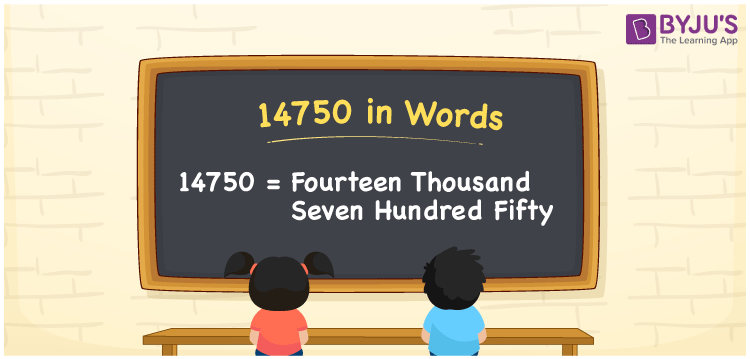# 14750 in Words

14750 in words is Fourteen Thousand Seven Hundred Fifty. For example, if you earned Rs. 14750 in a month, you can write, “I have earned Rs. Fourteen Thousand Seven Hundred Fifty in a month”. In general, the number name of any number can be written using the ones, tens, hundreds, and thousands place of a number. Thus, the place value chart is useful for writing the number 14750 in words.

 14750 in words Fourteen Thousand Seven Hundred Fifty Fourteen Thousand Seven Hundred Fifty in Numbers 14750

## 14750 in English Words## How to Write 14750 in Words?

We can convert 14750 to words using a place value chart. This can be done as follows. The number 14750 has 5 digits, so let’s make a chart that shows the place value up to 5 digits.

 Ten thousands Thousands Hundreds Tens Ones 1 4 7 5 0

Thus, we can write the expanded form as:

1 × Ten thousand + 4 × Thousand + 7 × Hundred + 5 × Ten + 0 × One

= 1 × 10000 + 4 × 1000 + 7 × 100 + 5 × 10 + 0 × 1

= 14750

= Fourteen Thousand Seven Hundred Fifty

14750 is the natural number that is succeeded by 14749 and preceded by 14751.

14750 in words – Fourteen Thousand Seven Hundred Fifty

Is 14750 an odd number? – No.

Is 14750 an even number? – Yes

Is 14750 a perfect square number? – No

Is 14750 a perfect cube number? – No

Is 14750 a prime number? – No

Is 14750 a composite number? – Yes

## Frequently Asked Questions (FAQs) on 14750 in Words

Q1

### How to write 14750 in words?

14750 in English is written as “Fourteen Thousand Seven Hundred Fifty”.
Q2

### Is the number 14750 an odd number?

No, the number 14750 is not an odd number.
Q3

### Is 14750 a composite number?

Yes, 14750 is a composite number.
Q4

### What is the place value of 7 in the number 14750?

The place value of 7 in 14750 is 7×100 = 700.
Q5

### What is the face value of 7 in the number 14750?

The face value of 7 in the number 14750 is 7 only.
Q6

### What is the product of the place value of 5 and the place value of 7 in 14750?

The place value of 5 is 5х10= 50 and the place value of 7 is 7х100=700
Therefore, product = 50 х 700 = 35,000